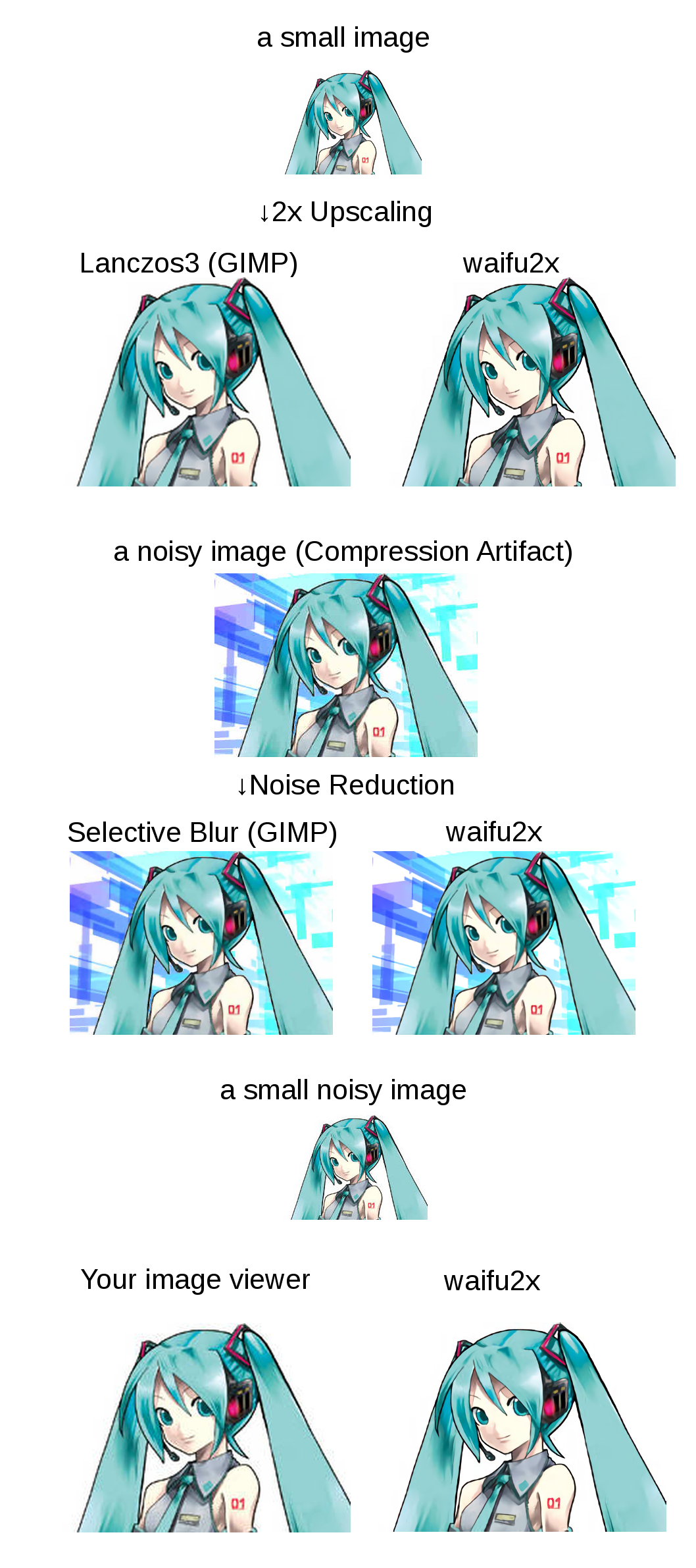# 并行与分布式计算（1）

## 课后作业

Write an essay describing a research problem in your major that would benefit from the use of parallel computing. Provide a rough outline of how parallelism would be used. Would you use task- or data-parallelism?

1. 能否将工作分解成为方便同时处理的离散成分；
2. 能否随时并且及时的执行多个程序指令；
3. 多处理单元解决该问题的耗时小于单处理单元。Assume that you have the followiog mix of instructions with average CPIs:

% of Mix Average CPI
ALU 47% 6.7
Branch 20% 5.0
Store 14% 7.1

The dock rate for this machine is 1 GHz.

You want to improve the performance of this machine, and are considering redesigning your multiplier to reduce the average CPI of multiply instructions. (Digress - why do multiplies take longer than adds?) If you make this change, the CPI of multiply instructions would drop to 6 (from 8). The percentage of ALU instructions that are multiply instructions is 23%. How much will perlormance improve by?

ALU 除了乘法之外部分的平均 CPI：$(6.7-8\times 23\%)/77\%\approx 6.3$